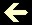PI VALUE

We can get PI value through the formula

2(2²÷1x3)(4²÷3x5)(6²÷5x7)(8²÷7x9)(10²÷9x11)... and so far

equivalent to 2(4÷3)(16÷15)(36÷35)(64÷63)(100÷99)...

which results

3,1415926535897932... and so far

If we run a thousand terms, we get only PI=3,14 and error from the third decimal place. Although we run a million terms

2(4÷3)(16÷15)(36÷35)(64÷63)(100÷99)... (4000000000000„3999999999999)

we get a poor answer, PI=3,14159 and error from the sixth decimal place. Check it. Input a number (from 1 to a million). Don't exceed a million, because you'll get a slow answer:

 N║ of terms PI Value